﻿

SpreadsheetGear enables ASP.NET, Windows Forms, WPF, iOS, Android, Linux, macOS, UWP and Silverlight developers to easily take advantage of scalable Excel Reporting, dynamic dashboards from Excel charts and ranges, powerful spreadsheet controls, comprehensive Excel compatible charting, the fastest and most complete Excel compatible calculations and more.

General Features

• Royalty free deployment to 32 bit and 64 bit Windows 2000, Windows XP, Windows Vista, Windows 7, Windows 8, Windows 8.1, Windows 10, Windows Server 2003 (including R2), Windows Server 2008 (including R2), Windows Server 2012 (including R2) and Windows Server 2016.
• Create, read, modify, view, edit, format, calculate, print and write Microsoft Excel 97-2003 (xls) and Excel 2007-2016 Open XML (xlsx and xlsm) workbooks without Excel.
• Scalable and reliable Excel Reporting.
• Powerful Windows Forms, WPF and Silverlight spreadsheet controls.
• Fastest and most complete Excel compatible calculation engine available (significantly faster than Excel in many cases).
• Easily create images from Excel charts and ranges.
• Comprehensive Excel compatible charting.
• Versatile autofilters with top or bottom n items or percent, above or below average, custom criteria, sorting, SUBTOTAL and more.
• Read and write password protected xls, xlsx and xlsm workbooks, including new Agile Encryption used by Excel 2013 and later.
• Read and write CSV and tab separated text files.
• Support for cell comments, pictures, text boxes, check boxes, drop-downs, list boxes, spinners, scrollbars, buttons, lines and many autoshapes.
• Drag Fill / Auto Fill including support for numbers, dates, times, month names, day names and certain text patterns.
• Fill Data Series support including automatic, chronological, linear, growth, day, weekday, month, year, start value, step value, stop value and the ability to use regression for linear or exponential trends.
• Fast single variable and single target goal seeking.
• Data validation including operators, alert messages, alert styles, custom formulas and drop-down lists.
• Conditional formats with support for simple comparison operators and custom formulas.
• Copy and insert DataTables into pre-formatted ranges with complete formula, border and format fixups.
• Easily convert entire workbooks or specific ranges to a DataSet or DataTable.
• Copy, transpose and consolidate across cells, worksheets, workbooks and workbook sets.
• Insert, delete and clear rows, columns and cells.
• Stable sort with an unlimited number of keys and the ability to sort text as numbers.
• Grouping and outlining of rows and columns.
• Supports 1,048,576 rows, 16,384 columns, 64K worksheets, unlimited workbooks and unlimited workbook sets.
• Copy, insert and move entire worksheets.
• Reads and writes VBA Macros.
• A wide variety of C# and Visual Basic samples including live ASP.NET and Silverlight samples.

Calculation Engine

• Fastest and most complete Excel compatible calculation engine available (significantly faster than Excel in many cases).
• Multithreaded calculations efficiently utilize todays multi-core CPUs.
• Interruptible background calculation enables responsive applications.
• 449 Excel compatible financial, date, time, text, lookup, math, trigonometry, statistical, engineering, database, information, logical and web functions.
• All types, operators, defined names, data tables and arrays.
• Support for 64K worksheets and unlimited workbooks in formulas.
• Worksheet specific defined names and global defined names.
• Custom functions, minimal recalc, iteration, precision as displayed and more.
• Copy formulas between cells, worksheets, workbooks and workbook sets with full support for reference fixups.
• Insert and delete worksheets, rows, columns and cells with full support for reference fixups.
• Cut, copy and paste with full support for smart reference fixups across multiple worksheets and workbooks.
• Special care was taken with numerical stability.

Workbook Features

• Read and write workbooks to disk, memory streams and byte arrays.
• Read and write password protected xls, xlsx and xlsm workbooks, including new Agile Encryption used by Excel 2013 and later.
• Workbook and worksheet scoped named ranges.
• Options to show and hide worksheet tabs and scrollbars.

Worksheet Features

• AutoFilters
• Top or bottom n items.
• Top or bottom n percent.
• Above or below average.
• Last day, month, quarter, week or year.
• This day, month, quarter, week or year.
• Next day, month, quarter, week or year.
• Specific day, month, year, hour, minute or second.
• Year to date.
• Filter by font color or interior color.
• Custom criteria such as " > 50 ".
• Filter by specific list of values.
• Support for the SUBTOTAL function which works in conjunction with autofilters to return various statistics for filtered items including average, count, max, min, product, standard deviation, sum and variance.
• Autofilter support in many commands including cell formatting, copy paste, fill, delete and more.
• Drag Fill / AutoFill, including suppor for numbers, dates, times, month names, day names and certain text patterns.
• Fill Data Series support including automatic, chronological, linear, growth, day, weekday, month, year, start value, step value, stop value and the ability to use regression for linear or exponential trends.
• GoalSeek - Fast single variable and single target goal seeking.
• Cell Data Validation - including operators, alert messages, alert styles, custom formulas and drop-down lists.
• Conditional Formatting
• Simple comparison operators
• Custom formulas
• Icon Sets
• Data Bars
• Color Scales
• Highlight Cells
• Top n / Bottom n Rules
• Overlapping Rules
• ADO.NET DataSet and DataTable Conversion
• Supports 1,048,576 rows, 16,384 columns, 64K worksheets, unlimited workbooks and unlimited workbook sets.
• Insert, delete and clear rows, columns and cells.
• Enable or disable worksheet protection, worksheet passwords and cell locking.
• Autoshapes, text boxes and pictures including JPEG, PNG, GIF, BMP, WMF and EMF.

Printing Features

• Print workbooks, worksheets, ranges and charts in any combination.
• Horizontal and vertical page breaks.
• Page Setup Options
• Print area, title rows and title columns.
• Margins, page order, page orientation, zoom and fit to pages.
• Print preview.
• Print to XPS files.

Charting Features

• The most comprehensive Excel compatible charting support available in a Microsoft .NET Framework component.
• GDI+ and XAML imaging classes provide the ability to generate an image (such as GIF or PNG) with one line of code.
• Chart rendering supports the most common charting features:
• Chart types include area, bar, column, line, pie, stock, XY scatter, radar and bubble charts.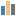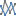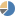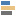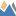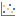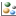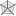• Chart type options include multiple chart groups (combination charts), stacked and 100% stacked values, bar gap and overlap, line markers, drop lines, high-low lines, trendlines, series line smoothing, open-close bars, error bars, exploded pies, and data point labels.
• Axes options include axes titles, primary and secondary axes sets, auto and manual scaling, logarithmic and date scales, major and minor gridlines and tick marks, reversed axes, tick label positions and rotated text.
• General chart options include chart titles, legends, theme colors, auto colors, gradients including Excel compatible preset gradients, solid and dashed line styles, number formats and fonts.

Formatting Features

• Number formats, including full support for getting and setting Excel compatible custom number formats as well as getting the formatted text of a cell as Excel would display it.
• Fonts, borders, Office theme colors with tinting and shading, RGB colors, Excel palette indexed colors and patterns.
• Horizontal alignment, vertical alignment, word wrap and rotated text.
• Merged cells, hyperlinks and conditional formats.
• Grouping and outlining of rows and columns.
• Automatic row heights and column widths.
• Copy formats without affecting formulas or values.
• Named cell style support.

• Windows Forms, WPF and Silverlight WorkbookView spreadsheet controls provide viewing, navigation and editing support which is familiar to Excel users.
• In-cell editing and optional FormulaBar control provide the ability to enter and edit cell values and formulas, including support for pointing at cell references in formulas across worksheets and workbooks.
• Smart data entry with optional data validation as well as automatic recognition and formatting of dates, times, percentages, currency, scientific notation and fractions will be familiar to Excel users.
• Conditional formatting enables font colors, font styles, background colors, borders as well as other graphical features such icon sets, data bars, color scales and more, which are based on simple comparison operators, complex formulas or more advanced rules.
• Grouping and outlining which allows users to easily expand and collapse the displayed level of detail.
• WorkbookDesigner provides an MDI Windows application which may be used from within Visual Studio 2005, Visual Studio 2008, Visual Studio 2010, Visual Studio 2012, Visual Studio 2013, Visual Studio 2015 and Visual Studio 2017 at design time and by your applications at run time.
• WorkbookExplorer provides the ability to manipulate workbooks, worksheets and defined names as well as their properties.
• RangeExplorer provides extensive modeless formatting of the currently selected range with support for number formats, alignment, fonts, borders, colors, patterns, gradients, protection options, hyperlinks, conditional formats and data validation.
• ChartExplorer provides basic modeless modification and formatting of the selected chart with support for chart types and data sources, legend options, primary and secondary axes options and various series options.
• Modeless find and replace dialog with support for find, find all, replace and replace all.
• Support for an unlimited number of workbooks associated with each WorkbookView.
• DisplayReference and DisplayReferenceName properties allow complete control over what appears for each sheet tab, including the ability to make multiple workbooks appear as one workbook and the ability to specify dynamically changing ranges using worksheet functions such as OFFSET().
• Cut, copy, paste and paste special support including rich data and formatting interchange with Excel and full formula fixups.
• Frozen rows and columns, split panes, sheet tab colors, zoom, multi-level undo/redo, autofit rows and columns, goto, clear, printing, print preview, interruptible background calculation, real time workbook updates and more.
• CommandManager provides the ability to add custom undoable commands as well as the ability to override the behavior of common commands.
• Full integration with Visual Studio 2005, Visual Studio 2008, Visual Studio 2010, Visual Studio 2012, Visual Studio 2015 and Visual Studio 2017, including the ability to use the WorkbookDesigner, WorkbookExplorer, RangeExplorer, ChartExplorer and ShapeExplorer at design time and at runtime.
• SpreadsheetGear Explorer Sample Solutions for Windows and Silverlight provide source code which demonstrates commonly used features and APIs.

Supported File Formats

• XLSX - Open XML Workbook
• XLSM - Open XML Macro Enabled Workbook
• XLS - Excel 97-2003 Workbook
• CSV - Comma Delimited Text
• TXT - Tab Delimited Text

Microsoft .NET Framework Features

• Easy to use API which will be familiar to Excel VBA and COM developers while taking advantage of .NET's expressiveness.
• Supports 32 and 64 bit versions of the Microsoft .NET Framework 2.0, .NET 3.0, .NET 3.5 .NET 4.0, .NET 4.5, .NET 4.6, .NET 4.7 and Silverlight 5.
• Supports 32 and 64 bit versions of Windows 2000, Windows XP, Windows Vista, Windows 7, Windows 8, Windows 8.1, Windows 10, Windows Server 2003 (including R2), Windows Server 2008 (including R2), Windows Server 2012 (including R2) and Windows Server 2016.
• Microsoft Visual Studio 2005, Visual Studio 2008, Visual Studio 2010, Visual Studio 2012, Visual Studio 2013, Visual Studio 2015 and Visual Studio 2017 integration, including IntelliSense and Dynamic Help.
• The security of 100 percent safe managed code which does not require FullTrust.
• Hassle free deployment with strong named assemblies as well as no license keys, registry entries or configuration issues.
• No dependency on Microsoft Excel, which is not supported in a server environment.

Supported Excel Compatible Functions

449 of Excel 2016's financial, date, time, text, lookup, math, trigonometry, statistical, engineering, database, information, logical and web functions.

Database and List Management Functions

 DAVERAGE Indicates the average of the values that meet the specified criteria. DCOUNT Counts the number of cells containing numbers that meet the specified criteria. DCOUNTA Counts nonblank cells containing numbers or text that meet the specified criteria. DGET Returns a single value that meets the specified criteria. DMAX Extracts the highest value that meets the specified criteria. DMIN Extracts the lowest value that meets the specified criteria. DPRODUCT Returns the product of the values that meet the specified criteria. DSTDEV Estimates the standard deviation of a population, based on a sample of selected entries from the database. DSTDEVP Returns the calculation of the standard deviation of a population, based on the sum of the whole population. DSUM Returns the total of the values that meet the specified criteria. DVAR Estimates the variance of a sample population based on the values that meet the specified criteria. DVARP Returns the calculation of the true variance of an entire population based on the values that meet the specified criteria.

Date and Time Functions

 DATE Returns the serial number that represents a date. DATEDIF Returns the difference of two dates in years, months or days. DATEVALUE Converts date text to a DATEVALUE serial number. DAY Returns the corresponding day of the month serial number or date text from 1 to 31. DAYS Returns the number of days between the two specified dates. DAYS360 Returns the number of days between two set dates based on a 360-day year. EDATE Returns the value or serial number of the date which is a certain number of months before or after a user-specified date. EOMONTH Returns the date at the end of the month a specified number of months before or after a specified date. HOUR Returns the hour as a serial number integer between 0 and 23. ISOWEEKNUM Returns the ISO week number for a specified date. MINUTE Returns the serial number that corresponds to the minute. MONTH Returns the corresponding serial number of the month of a date between 1 and 12. NETWORKDAYS Returns the number of working days between two dates. Excludes weekends and specified holidays. NETWORKDAYS.INTL Returns the number of whole workdays between two dates using parameters to indicate which and how many days are weekend days. NOW Returns the current date and time in the form of a serial number. SECOND Returns the seconds portion of a serial time value. TIME Returns the decimal value of a given time. TIMEVALUE Returns the decimal number for a given time. TODAY Returns the current date as a serial number. WEEKDAY Returns the corresponding day of the week as a serial number. WEEKNUM Returns the number where a week falls numerically within a year. WORKDAY Returns a date that is a specified number of working days before or after a given date. WORKDAY.INTL Returns the serial number of the date before or after a specified number of workdays using parameters to indicate which and how many days are weekend days. YEAR Returns the corresponding year as a serial number in the form of an integer. YEARFRAC Calculates the fraction of the year between two dates.

Engineering Functions

 BESSELI Returns the BESSEL function in modified form for imaginary arguments. BESSELJ Returns the actual BESSEL function. BESSELK Returns the BESSEL function in modified form for imaginary arguments. BESSELY Returns the BESSEL function, also known as the Weber or Neumann function. BIN2DEC Converts a binary number to decimal form. BIN2HEX Converts a binary number to a hexadecimal. BIN2OCT Converts a binary number to octal form. BITAND Returns the bitwise AND of the two specified numbers. BITLSHIFT Returns the specified number shifted left by the specified amount. BITOR Returns the bitwise OR of the two specified numbers. BITRSHIFT Returns the specified number shifted right by the specified amount. BITXOR Returns the bitwise XOR of the two specified numbers. COMPLEX Converts real and imaginary coefficients into a complex number of the form x + yi or x + yj. CONVERT Interprets data from one measurement system to another. DEC2BIN Converts decimal numbers to binary form. DEC2HEX Converts decimal numbers to hexadecimal. DEC2OCT Converts decimal numbers to octal. DELTA Tests whether numbers or values are equal with a number result. Returns "0" for unequal, "1" for equal. ERF Returns the integrated error function between a lower and upper limit. ERF.PRECISE Returns the error function ERFC Returns a complementary ERF function integrated between 'x' and infinity. ERFC.PRECISE Returns the complementary ERF function integrated between x and infinity GESTEP Returns the value 1 if the number is greater than or equal to a specified step value, otherwise it returns 0. HEX2BIN Converts hexadecimal numbers to binary form. HEX2DEC Converts hexadecimal numbers to decimal form. HEX2OCT Converts hexadecimal numbers to octal form. IMABS Returns the absolute value (modulus) of a complex number in x+yi or x+yj text format. IMAGINARY Returns the coefficient of a complex number in x+yi or x+yj text format. IMARGUMENT Returns the theta argument - an angle expressed in radians. IMCONJUGATE Returns the complex conjugate of a complex number in x+yi or x+yj text format. IMCOS Returns the cosine of a complex number in x+yi or x+yj text format. IMCOSH Returns the hyperbolic cosine of the specified complex number. IMCOT Returns the cotangent of the specified complex number. IMCSC Returns the cosecant of the specified complex number. IMCSCH Returns the hyperbolic cosecant of the specified complex number. IMDIV Returns the quotient of complex numbers in x+yi or x+yj text format. IMEXP Returns the exponential of a complex number in x+yi or x+yj text format. IMLN Returns the natural logarithm of a complex number in x+yi or x+yj text format. IMLOG10 Returns the common logarithm (Base 10) of a complex number in x+yi or x+yj text format. IMLOG2 Returns the common logarithm (Base 2) of a complex number in x+yi or x+yj text format. IMPOWER Returns a complex number raised to a power in x+yi or x+yj text format. IMPRODUCT Returns the product from 2 to 29 complex numbers in x+yi or x+yj text format. IMREAL Returns the real coefficient of a complex number in x+yi or x+yj text format. IMSEC Returns the secant of the specified complex number. IMSECH Returns the hyperbolic secant of the specified complex number. IMSIN Returns the sine of a complex number in x+yi or x+yj text format. IMSINH Returns the hyperbolic sine of the specified complex number. IMSQRT Returns the square root of a complex number in x+yi or x+yj text format. IMSUB Returns the difference of two complex numbers in x+yi or x+yj text format. IMSUM Returns the sum of 2 to 29 complex numbers in x+yi or x+yj text format. IMTAN Returns the tangent of the specified complex number. OCT2BIN Converts an octal number to binary form. OCT2DEC Converts an octal number to decimal form. OCT2HEX Converts an octal number to hexadecimal form.

Financial Functions

 ACCRINT Returns accrued interest for securities that pay periodic interest. ACCRINTM Returns the accrued interest for securities that pay interest at the maturity date. AMORDEGRC Returns the depreciation for each accounting period within the formula. AMORLINC Returns the depreciation for each accounting period. COUPDAYBS Returns the number of days from the beginning of the period to the coupon-period settlement date. COUPDAYS Returns the number of days in the period that contains the coupon period settlement date. COUPDAYSNC Returns the number of days between the settlement date to the next coupon date. COUPNCD Returns the next coupon date after the settlement date. COUPNUM Returns the total number of coupons to be paid between the settlement and maturity dates, rounded up to the nearest whole coupon. COUPPCD Returns the coupon date previous to the settlement date. CUMIPMT Returns the cumulative interest on a loan between start and stop dates. CUMPRINC Returns the cumulative principal amount between start and stop dates on a loan or mortgage. DB Returns the asset depreciation for a period using the fixed declining balance method. DDB Returns the asset depreciation for a period using the double-declining balance method or another specified method. DISC Returns the security discount rate. DOLLARDE Converts a fraction dollar price into a decimal dollar price. DOLLARFR Converts a decimal dollar price into a fraction dollar price. DURATION Returns the Macauley duration for an assumed par value. EFFECT Returns the effective interest rate annually. This is based on the nominal annual interest rate and the number of compounding periods per year. FV Returns the future value of an investment that makes payments as a lump sum or as a series of equal periodic payments. FVSCHEDULE Returns the future value of a principal amount after applying several, or a series of compound interest rates. INTRATE Returns the interest rate of a security that is fully invested. IPMT Returns the interest for a period of time based on an investment with periodic constant payments and a constant interest rate. IRR Returns the internal rate of return for a series of cash flows represented by numbers in the form of values. ISPMT Calculates the interest paid during a defined period of an investment. MDURATION Returns the modified duration of a security with a par value assumed to be \$100. MIRR Returns a modified internal rate of return for several periodic cash flows. NOMINAL Returns the nominal annual interest rate given an effective rate and the total number of compounding periods for the year. NPER Returns the total number of periods for an investment. This is based on a periodic constant payment and a constant interest rate. NPV Calculates the net present value of an investment from the discount rate and several future payments and income. ODDFPRICE Returns the value of a security based on a per \$100 face value and an odd (short or long) first period. ODDFYIELD Returns the security yield with an odd first period. ODDLPRICE Returns the per \$100 face value of a security having an odd last coupon period. ODDLYIELD Returns the security yield that has an odd last period. PDURATION Returns the number of periods for the specified present value to reach the specified future value given the specified interest rate. PMT Calculates the loan payment for a loan based on constant payments and constant interest rates. PPMT Returns the principal payment for a period of an investment based on periodic constant payments and a constant interest rate. PRICE Returns the value of a security based on price per \$100 face value and periodic interest payments. PRICEDISC Returns the value of a discounted security based on a price per \$100 face value. PRICEMAT Returns the value of a security that pays interest at maturity and price per \$100 face value. PV Returns the present value based on an investment. RATE Returns per period the interest of an annuity. RECEIVED Based on a fully invested security, returns the amount received at maturity. RRI Returns the effective interest rate required for the specified present value to reach the specified future value in the specified number of periods. SLN Returns the straight-line depreciation on an asset. SYD Based on a specified period, SYD returns the sum-of-years' digits depreciation of an asset. TBILLEQ Returns the bond equivalent yield for a treasury bill. TBILLPRICE Returns the price per \$100 face value for a treasury bill. TBILLYIELD Returns the yield of a treasury bill. VDB For a period you specify, returns the depreciation of an asset. XIRR Returns the internal rate of return for a schedule of cash flows that is not necessarily periodic. XNPV Returns the net present value for a schedule of cash flows that is not necessarily periodic. YIELD Based on a yield that pays periodic interest, returns the yeild of the security. YIELDDISC Returns the annual yield for a discounted security. YIELDMAT Returns the annual yield based on a security that pays interest at a maturity.

Information Functions

 CELL Returns information about a cell's location, formatting, or contents in the upper-left cell in a reference. ERROR.TYPE Returns the corresponding number value associated with an error type in Microsoft Excel. INFO Returns operating environment information. ISBLANK Returns TRUE if the cell is empty, FALSE if it contains data. ISERR Returns TRUE if value contains any error value except #N/A, FALSE if it does not. ISERROR Returns TRUE if value contains any error value (including #N/A), FALSE if it does not. ISEVEN Returns TRUE if value is an even number, FALSE if it is not. ISFORMULA Returns TRUE if the specified cell contains a formula. ISLOGICAL Returns TRUE if value is a logical value, FALSE if it is not. ISNA Returns TRUE if value is #N/A, FALSE if it is not. ISNONTEXT Returns TRUE if value is not text, FALSE if it is. ISNUMBER Returns TRUE if value is a number, FALSE if it is not. ISODD Returns TRUE if value is an odd number, FALSE if it is not. ISREF Returns TRUE if value is a reference, FALSE if it is not. ISTEXT Returns TRUE if value is text, FALSE if it is not. N Returns a value converted to a number. NA An alternative representation of the error value #N/A. SHEET Returns the one based index of the specified sheet, or the index of the sheet containing the formula if no sheet is specified. SHEETS Returns the number of sheets in a 3d cell reference, or the number of sheets in the workbook containing the formula if no reference is specified. TYPE Determines the type of value in a cell.

Logical Functions

 AND Returns TRUE if all the arguments are TRUE in the formula, and FALSE if any one argument is FALSE. FALSE Returns the value FALSE. May be typed directly into the cell as "FALSE". IF Returns a value if one condition is TRUE and returns another value if the condition is FALSE. IFERROR Returns a value you specify if a formula evaluates to an error; otherwise, returns the result of the formula IFNA Returns the specified first argument unless it is #N/A, in which case it returns the specified second argument. NOT Returns the reverse value of its arguments; TRUE becomes FALSE and FALSE becomes TRUE. OR Returns FALSE if all arguments are FALSE, and TRUE if at least one argument is TRUE. TRUE Returns the value TRUE. May be typed directly into the cell as "TRUE". XOR Returns TRUE if the specified arguments contain an odd number of TRUE values, or FALSE if the values contain an even number of TRUE values.

Lookup and Reference Functions

 ADDRESS Given specified row and column numbers, creates a cell address as text. AREAS Returns the number of areas based on a reference. CHOOSE Returns an item from a list of values. COLUMN Returns the column number(s) based on a given reference. COLUMNS Returns the number of columns based on an array or reference. HLOOKUP Searches for a specified value in an array or a table's top row. HYPERLINK Creates a shortcut to jump to a document stored on a network server. INDEX Returns the value of an element selected by the row number and column letter indexes. INDIRECT Returns the contents of a cell using its reference. LOOKUP Looks in the first row or column of a range or array, and returns the specified value from the same position in the last row or column of the range or array. MATCH Returns the relative position of an item in an array that matches a specified value in a specified order, or the position of an item. OFFSET Returns a reference to a range that is a specific number of rows and columns from a cell or range of cells. ROW Returns the row number based on a reference. ROWS Returns the number of rows in a reference or array. TRANSPOSE Returns a horizontal range of cells as vertical or vice versa. VLOOKUP Searches for a value in the leftmost column of a table and returns a value from the same row in a column number that you specify.

Math and Trigonometry Functions

 ABS Returns the absolute value of a number. ACOS Returns the arccosine of a number in radians in the range 0 to pi. ACOSH Returns the inverse hyperbolic cosine of a number. ACOT Returns the inverse cotangent of the specified number. ACOTH Returns the inverse hyperbolic cotangent of the specified number. AGGREGATE Returns an aggregate in a list or database ARABIC Converts the specified Roman numeral to a number. ASIN Returns the arcsine of a number in radians in the range -pi/2 to pi/2. ASINH Returns the inverse hyperbolic sine of a number. ATAN Returns the arctangent of a number in radians in the range -pi/2 to pi/2 ATAN2 Returns the four-quadrant arctangent of the specified x- and y- coordinates in radians between -pi and pi excluding -pi. A positive result represents a counterclockwise angle from the x-axis, a negative result represents a clockwise angle. ATANH Returns the inverse hyperbolic tangent of a number. BASE Converts the specified number to text with the specified radix and minimum length. CEILING Returns a number rounded up, away from zero, to the nearest multiple of significance. CEILING.MATH Returns the specified number rounded up using the specified significance and mode. CEILING.PRECISE Rounds a number to the nearest integer or to the nearest multiple of significance. Regardless of the sign of the number, the number if rounded up. COMBIN Returns the number of combinations for a given number of items. COMBINA Returns the number of combinations with the specified number of items. COS Returns the cosine of the given angle. COSH Returns the hyperbolic cosine of a number. COT Returns the cotangent of the specified angle. COTH Returns the hyperbolic cotangent of the specified angle. CSC Returns the cosecant of the specified angle. CSCH Returns the hyperbolic cosecant of the specified angle. DECIMAL Converts the specified text to a number using the specified radix. DEGREES Converts radians into degrees. EVEN Returns a number rounded up to the next even integer for positive integers and rounded down to the next even integer for negative numbers. EXP Returns e (2.71828182845804) raised to the power of a specified number. FACT Returns the factorial of a number. FACTDOUBLE Returns the double factorial of a number. FLOOR Returns a number rounded down, toward zero, to the nearest multiple of significance. FLOOR.MATH Returns the specified number rounded down using the specified significance and mode. FLOOR.PRECISE Rounds a number to the nearest integeror to the nearest multiple of significance. Regardless of the sign of the number, the number is rounded up. GCD Returns the greatest common divisor of two or more integers. INT Rounds a number down to the nearest integer. ISO.CEILING Returns the specified number rounded up using the specified significance. LCM Returns the least common multiple of integers. LN Returns the natural (base e) logarithm of a number. LOG Returns the logarithm of a number of the base you specify. LOG10 Returns the base-10 logarithm of a number. MDETERM Returns the matrix determinant of an array. MINVERSE Returns the inverse matrix for the matrix stored in an array. MMULT Returns the matrix product of two arrays. The result is an array with the same number of rows as array1 and the same number of columns as array2. MOD Returns the remainder of a division operation (modulus). MROUND Returns a number rounded to the desired multiple. Rounds up if the remainder after dividing the number by the multiple is at least half the value of the multiple. MULTINOMIAL Returns the ratio of the factorial of the sum of the values to the product of the factorials. MUNIT Returns an identity matrix with the specified n by n dimension. ODD Returns a number rounded up away from zero to the nearest odd integer. PI Returns the approximate number 3.14159265358979, the mathematical constant pi, accurate to 15 digits. POWER Returns the result of a specified number raised to a specified power. PRODUCT Multiplies all the numbers given as arguments and returns the product. QUOTIENT Returns the integer portion of a division. RADIANS Converts degrees to radians. RAND Returns an evenly distributed random number greater than or equal to 0 and less than 1. A new random number is returned every time the worksheet is calculated. RANDBETWEEN Returns a random integer between the integers you specify. A new random number is returned every time the worksheet is calculated. ROMAN Converts an Arabic numeral to Roman, as text. ROUND Round a number to a specified number of digits. ROUNDDOWN Rounds a number down, towards zero. ROUNDUP Rounds a number up, away from zero. SEC Returns the secant of the specified angle. SECH Returns the hyperbolic secant of the specified angle. SERIESSUM Returns the sum of a power series. SIGN Determines the sign of a number. Returns 1 if the value is positive, 0 if the value is 0, and -1 if the value is negative. SIN Returns the sine of a given angle. SINH Returns the hyperbolic sine of a number. SQRT Returns a positive square root. SQRTPI Returns the square root of (NUMBER * Pi) SUBTOTAL Returns a subtotal in a list or database. SUM Adds all the numbers in a range of cells. SUMIF Adds the cells specified by a certain criteria. SUMIFS Adds the cells in a range that meet multiple criteria SUMPRODUCT Multiplies corresponding components in the given arrays, and returns the sum of those products. SUMSQ Returns the sum of the squares of the arguments. SUMX2MY2 Returns the sum of the difference of squares of corresponding values in two arrays. SUMX2PY2 Returns the sum of the sum of squares of corresponding values in two arrays. SUMXMY2 Returns the sum of squares of differences of corresponding values in two arrays. TAN Returns the tangent of the given angle. TANH Returns the hyperbolic tangent of a number. TRUNC Truncates a number to an integer by removing the fractional part of a number.

Pre-Excel 2010 Statistical Functions

 BETADIST Returns the cumulative beta probability density function. BETAINV Returns the inverse of the cumulative beta probability density function. BINOMDIST Returns the individual term binomial distribution probability. CHIDIST Returns the one-tailed probability of the chi-squared (X^2) distribution; the area in the right tail under the chi-squared distribution curve. CHIINV Returns the inverse of the one-tailed probability of the chi-squared (X^2) distribution. CHITEST Returns the test for independence of the characteristics in a table. CONFIDENCE Returns the confidence interval for a population mean. COVAR Returns the covariance, the average of products of deviations, for each data point pair. EXPONDIST Returns the exponential distribution. FDIST Returns the F probability distribution. FINV Returns the inverse of the F probability distribution. FTEST Returns the result of an F-test. GAMMADIST Returns the gamma distribution. GAMMAINV Returns the inverse of the gamma cumulative distribution. LOGINV Returns the inverse of the lognormal cumulative distribution function of x, where ln(x) is normally distributed with parameters mean and standard deviation. LOGNORMDIST Returns the cumulative lognormal distribution of x, where ln(x) is normally distributed with parameters mean and standard deviation. MODE Returns the most frequently occuring, or repetitive, number in an array or range of data. NEGBINOMDIST Returns the negative binomial distribution. NORMDIST Returns the normal cumulative distribution for the specified mean and standard deviation. NORMINV Returns the inverse of the normal cumulative distribution for the specified mean and standard deviation. NORMSDIST Returns the standard normal cumulative distribution function. PERCENTILE Returns the k-th percentile of values in a range. PERCENTRANK Returns the rank of a value in a data set set as a percentage of the data set. POISSON Returns the Poisson distribution. QUARTILE Returns the quartile of a data set. RANK Returns the rank of a number in a list of numbers. STDEV Estimates standard deviation based on a sample. STDEVP Estimates standard deviation based on a sample assuming that the arguments represent the total population. TDIST Returns the percentage points (probability) for the student t-distribution, where a numeric value (x) is a calculated value of t for which the percentage points are to be computed. TINV Returns the t-value of the Student's t-distribution as a function of the probability and the degrees of freedom. TTEST The probability associated with t-test. VAR Returns an estimate for the variance of a population based on a sample data set. VARP Calculates variance based on the entire population. WEIBULL Returns the Weibull distribution. ZTEST Returns the two-tailed P-value of a z-test.

Statistical Functions

 AVEDEV Retuns the average of the absolute deviations of data points from their mean. AVERAGE Returns the average of its arguments. AVERAGEA Returns the average of the values in its list of arguments including text and logical values. AVERAGEIF Returns the average (arithmetic mean) of all the cells in a range that meet a given criteria AVERAGEIFS Returns the average (arithmetic mean) of all cells that meet multiple criteria BETA.DIST Returns the beta cumulative distribution function BINOM.DIST.RANGE Returns the probability of the specified trial using a binomial distribution. BETA.INV Returns the inverse of the cumulative distribution function for a specified beta distribution BINOM.DIST Returns the individual term binomial distribution probability BINOM.INV Returns the smallest value for which the cumulative binomial distribution is less than or equal to a criterion value CHISQ.DIST Returns the chi-squared distribution CHISQ.DIST.RT Returns the one-tailed probability of the chi-squared distribution CHISQ.INV Returns the inverse of the left-tailed probability of the chi-squared distribution CHISQ.INV.RT Returns the inverse of the right-tailed probability of the chi-squared distribution CHISQ.TEST Returns the test for independence. CONFIDENCE.NORM Returns the confidence interval for a population mean. CONFIDENCE.T Returns the confidence interval for a population mean, using a Student's t distribution CORREL Returns the correlation coefficient between two data sets. COUNT Counts the number of cells that contain numbers (including dates and formulas that evaluate to numbers) within the list of arguments. COUNTA Counts the number of cells that are not empty. COUNTBLANK Counts the empty cells in a specified range. COUNTIF Counts the number of cells in a range that meet a given criteria. COUNTIFS Counts the number of cells within a range that meet multiple criteria COVARIANCE.P Returns covariance, the average of the products of paired deviations COVARIANCE.S Returns the sample covariance, the average of the products deviations for each data point pair intwo data sets CRITBINOM Returns the minimum number yields a binomial distribution less than or equal to the specified criteria DEVSQ Returns the sum of the squares of deviations of a data set from their sample mean. EXPON.DIST Returns the exponential distribution. F.DIST Returns the F probability distribution. F.DIST.RT Returns the (right-tailed) F probability distribution (degree of diversity) for two data sets F.INV Returns the inverse of the F probability distribution F.INV.RT Returnd the inverse of the (right-tailed) F probability distribution F.TEST Returns the result of an F-test. FISHER Returns the Fisher transformation at x. FISHERINV Returns the inverse of the Fisher transformation at y. FORECAST Calculates or predicts a future value by using existing values. FREQUENCY Calculates how often values occur within a range of values and then returns a vertical array of numbers. GAMMA Returns the gamma funnction result for the specified number. GAMMA.DIST Returns the gamma distribution. GAMMA.INV Returns the inverse of the gamma cumulative distribution. GAMMALN Returns the natural logarithm of the gamma function. GAMMALN.PRECISE Returns the natural logarithm of the gamma function. GAUSS Returns the probability that a number will fall between the mean and the specified standard deviation in a normal distribution. GEOMEAN Returns the geometric mean of an array or range of positive data. GROWTH Calculates predicted exponential growth by using existing data. HARMEAN Returns the harmonic mean of a data set. HYPGEOM.DIST Returns the hypergeometric distribution. HYPGEOMDIST Returns the hypergeometric distribution. INTERCEPT Calculates the point at which a line will intersect the y-axis by using existing x and y values. KURT Returns the Kurtosis of a data set. LARGE Returns the k-th largest value in a data set. LINEST Calculates a straight line that best fits your data using the least squares method. LOGEST Calculates an exponential curve that fits your data and returns an array of values that describes the curve. LOGNORM.DIST Returns the lognormal distribution, of x, where ln(x) is normally distributed with mean and standard deviation. LOGNORM.INV Returns the inverse of the lognormal cumulative distribution. MAX Returns the largest value in a set of values. MAXA Returns the largest value in a set of values including text and logical values. MEDIAN Returns the median of the given numbers. MIN Returns the smallest value in a set of values. MINA Returns the smallest value in a set of values including text and logical values. MODE.MULT Returns a vertical array of the most frequestly occurring, or repetitive values in an array or range of data. MODE.SNGL Returns the most common value in a data set. NEGBINOM.DIST Returns the negative binomial distribution. NORM.DIST Returns the normal cumulative distribution. NORM.INV Returns the inverse of the normal cumulative distribution. NORM.S.DIST Return the standard normal cumulative distribution. NORM.S.INV Returns the inverse of the standard normal cumulative distribution. NORMSINV Returns the inverse of the standard normal cumulative distribution function. PEARSON Returns the Pearson product moment correlation coefficient, r, a dimensionless index that ranges from -1.0 to 1.0 inclusive and reflects the extent of a linear relationship between two data sets. PERCENTILE.EXC Returns the k-th percentile of values in a range, where k is in the range 0..1, exclusive PERCENTILE.INC Returns the k-th percentile of values in a range. PERCENTRANK.EXC Returns the rank of a value in a data set as a percentage (0..1, exclusive) of the data set PERCENTRANK.INC Returns the percentage rank of a value in a data set PERMUT Returns the number of permutations for a given number of objects that can be selected from a range of numbers. PERMUTATIONA Returns the number of permutations given the specified total number of items and the specified number of items chosen for each permutation. PHI Returns the value of the probability density function of the specified number for the standard normal distribution. POISSON.DIST Returns the Poisson distribution. PROB Returns the probability that values in a range are between two specified limits. QUARTILE.EXC Returns the quartile of the data set, based on percentile values fro 0..1, exclusive. QUARTILE.INC Returns the quartile of a data set. RANK.AVG Returns the rank of a number in a list of numbers. RANK.EQ Returns the rank of a number in a list of numbers. RSQ Returns the r^2 value of a linear regression line. SKEW Returns the skew of a distribution. SKEW.P Returns the population skewness of the specified distribution. SLOPE Returns the slope of a regression line through data points in KNOWN_Y'S and KNOWN_X'S. SMALL Returns the k-th smallest value in a data set. STANDARDIZE Returns a normalized value from a distribution characterized by MEAN and STANDARD_DEV. STDEV.P Calculates standard deviation based on the entire population STDEV.S Estimates standard deviation based on a sample. STDEVA Estimates standard deviation based on a sample. Includes text and logical values. STDEVPA Estimates standard deviation based on a sample assuming that the arguments represent the total population. Includes text and logical values. STEYX Returns the standard error of the predicted y value for each x in the regression. T.DIST Returns the percentage points (probability) for the student t-distribution. T.DIST.2T Returns the percentage points (probability) for the student t-distribution. T.DIST.RT Returns the Student's t-distribution. T.INV Returns the t-value of the Student's t-distribution as a function of the probability and the degrees of freedom. T.INV.2T Returns the inverse of the Student's t-distribution. T.TEST Returns the probability associated with a Student's t-test. TREND Returns the y-values along a linear trendline that best fits the values in a data set. TRIMMEAN Returns the mean of the interior of a data set. VAR.P Calculates variance based on the entire population VAR.S Estimates variance based on a sample. VARA Returns an estimate for the variance of a population based on a sample data set and may include text or logical values. VARPA Calculates variance based on the entire population and may include text or logical values. WIEBULL.DIST Returns the Weibull distribution. Z.TEST Returns the one-tailed probability-value of a z-test.

Text Functions

 CHAR Returns the character specified by a number. CLEAN RRemoves all nonprintable characters from text. CODE Returns a numeric code from the first character in a text string. The opposite of the CHAR function. CONCATENATE Joins several text strings into one text string. DOLLAR Converts a number to text using Currency format, with the decimals rounded to the specified place. EXACT Compares two text strings and returns TRUE if they are exactly the same, and FALSE otherwise. FIND Locates one text string within another text string, and returns the number of the starting position of of FIND_TEXT from the leftmost character of WITHIN_TEXT. FINDB Returns the position of specified text within another specified text string based on the number of bytes each character uses from the first character of WITHIN_TEXT. FIXED Rounds a number to a specified number of decimals, formats the number in decimal format using a period and commas, and returns the result as text. LEFT Returns the first character(s) in a text string. LEFTB Returns the first character(s) in a text string based on a specified number of bytes LEN Returns the number of characters in a text string. LENB Returns the number of characters in a text string expressed in bytes. LOWER Converts all letters in a text string to lowercase. MID Returns a specific number of characters from a text string starting at the position you specify. MIDB Returns a group of characters based on a specified number of bytes from a text string starting at the position you specify. NUMBERVALUE Converts the specified text to a number using the specified decimal seperator and thousands separator. PROPER Capitalizes the first letter of each word in a text string or sentence. REPLACE Replaces part of a text string with a different text string based on the number of characters you specify. REPLACEB Replaces part of a text string with a different text string based on the number of characters you specify in terms of bytes. REPT Repeats specified text a given number of times. RIGHT Returns the last character(s) in a text string. RIGHTB Returns the last character(s) in a text string based on a specified number of bytes. SEARCH Returns the number of the character at which a specific character or text string is first found, reading from left to right. SEARCHB Returns the number of the character at which a specific character or text string is first found in bytes, reading from left to right. SUBSTITUTE Substitutes NEW_TEXT for OLD_TEXT in a string. T Returns the text referred to by a value. TEXT Converts a value to text in a specific number format. TRIM Removes all spaces from text except single spaces between words. UNICHAR Convert the specified UTF-32 code point to text. UNICODE Convert the first character in the specified text to a UTF-32 code point. UPPER Converts text to uppercase. USDOLLAR Converts a number to text using US Dollar format, with the decimals rounded to the specified place. VALUE Converts a text string that represents a number to a number.

Web Functions

 ENCODEURL Returns the specified string as an encoded URL. FILTERXML Returns the selected node(s) from the specified xml and xpath expression. WEBSERVICE Returns the text result of an HTTP request from the specified URL.

Which SpreadsheetGear Product is Right for You?

SpreadsheetGear 2017 for .NET comes with assemblies built for .NET 2.0 and .NET 4.0 while SpreadsheetGear for .NET Standard requires a platform which supports .NET Standard 1.3. SpreadsheetGear 2017 for Silverlight requires Silverlight 5. Although many features are identical, there are some differences...

ページトップへ